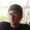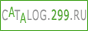–Ю–С–£–І–Х–Э–Ш–Х –Ю–Э–Ы–Р–Щ–Э –Ш –Т–Ш–Ф–Х–Ю–£–†–Ю–Ъ–ШMax Azimov–°–∞–љ–Ї—В-–Я–µ—В–µ—А–±—Г—А–≥–°—В–∞—В—М–Є –њ–Њ–ї—М–Ј–Њ–≤–∞—В–µ–ї—П–Т–Є–і–µ–Њ –њ–Њ–ї—М–Ј–Њ–≤–∞—В–µ–ї—ПPHP 5 Operators

PHP Operators

Operators are used to perform operations on variables and values.

PHP divides the operators in the following groups:
• Arithmetic operators
• Assignment operators
• Comparison operators
• Increment/Decrement operators
• Logical operators
• String operators
• Array operators

PHP Arithmetic Operators

The PHP arithmetic operators are used with numeric values to perform common arithmetical operations, such as addition, subtraction, multiplication etc.

Operator Name Example Result
+ Addition \$x + \$y Sum of \$x and \$y
- Subtraction \$x - \$y Difference of \$x and \$y
* Multiplication \$x * \$y Product of \$x and \$y
/ Division \$x / \$y Quotient of \$x and \$y
% Modulus \$x % \$y Remainder of \$x divided by \$y
** Exponentiation \$x ** \$y Result of raising \$x to the \$y'th power (Introduced in PHP 5.6)

## PHP Assignment Operators

The PHP assignment operators are used with numeric values to write a value to a variable.

The basic assignment operator in PHP is "=". It means that the left operand gets set to the value of the assignment expression on the right.

Assignment Same as... Description
x = y x = y The left operand gets set to the value of the expression on the right
x += y x = x + y Addition
x -= y x = x - y Subtraction
x *= y x = x * y Multiplication
x /= y x = x / y Division
x %= y x = x % y Modulus

## PHP Comparison Operators

The PHP comparison operators are used to compare two values (number or string):

Operator Name Example Result
== Equal \$x == \$y Returns true if \$x is equal to \$y
=== Identical \$x === \$y Returns true if \$x is equal to \$y, and they are of the same type
!= Not equal \$x != \$y Returns true if \$x is not equal to \$y
<> Not equal \$x <> \$y Returns true if \$x is not equal to \$y
!== Not identical \$x !== \$y Returns true if \$x is not equal to \$y, or they are not of the same type
> Greater than \$x > \$y Returns true if \$x is greater than \$y
< Less than \$x < \$y Returns true if \$x is less than \$y
>= Greater than or equal to \$x >= \$y Returns true if \$x is greater than or equal to \$y
<= Less than or equal to \$x <= \$y Returns true if \$x is less than or equal to \$y

## PHP Increment / Decrement Operators

The PHP increment operators are used to increment a variable's value.

The PHP decrement operators are used to decrement a variable's value.

Operator Name Description
++\$x Pre-increment Increments \$x by one, then returns \$x
\$x++ Post-increment Returns \$x, then increments \$x by one
--\$x Pre-decrement Decrements \$x by one, then returns \$x
\$x-- Post-decrement Returns \$x, then decrements \$x by one

## PHP Logical Operators

The PHP logical operators are used to combine conditional statements.

Operator Name Example Result
and And \$x and \$y True if both \$x and \$y are true
or Or \$x or \$y True if either \$x or \$y is true
xor Xor \$x xor \$y True if either \$x or \$y is true, but not both
&& And \$x && \$y True if both \$x and \$y are true
|| Or \$x || \$y True if either \$x or \$y is true
! Not !\$x True if \$x is not true

## PHP String Operators

PHP has two operators that are specially designed for strings.

Operator Name Example Result
. Concatenation \$txt1 . \$txt2 Concatenation of \$txt1 and \$txt2
.= Concatenation assignment \$txt1 .= \$txt2 Appends \$txt2 to \$txt1

## PHP Array Operators

The PHP array operators are used to compare arrays.

Operator Name Example Result
+ Union \$x + \$y Union of \$x and \$y
== Equality \$x == \$y Returns true if \$x and \$y have the same key/value pairs
=== Identity \$x === \$y Returns true if \$x and \$y have the same key/value pairs in the same order and of the same types
!= Inequality \$x != \$y Returns true if \$x is not equal to \$y
<> Inequality \$x <> \$y Returns true if \$x is not equal to \$y
!== Non-identity \$x !== \$y Returns true if \$x is not identical to \$y

Operator Name Example Result
+ Addition \$x + \$y Sum of \$x and \$y
- Subtraction \$x - \$y Difference of \$x and \$y
* Multiplication \$x * \$y Product of \$x and \$y
/ Division \$x / \$y Quotient of \$x and \$y
% Modulus \$x % \$y Remainder of \$x divided by \$y
** Exponentiation \$x ** \$y Result of raising \$x to the \$y'th power (Introduced in PHP 5.6)

## PHP Assignment Operators

The PHP assignment operators are used with numeric values to write a value to a variable.

The basic assignment operator in PHP is "=". It means that the left operand gets set to the value of the assignment expression on the right.

Assignment Same as... Description
x = y x = y The left operand gets set to the value of the expression on the right
x += y x = x + y Addition
x -= y x = x - y Subtraction
x *= y x = x * y Multiplication
x /= y x = x / y Division
x %= y x = x % y Modulus

## PHP Comparison Operators

The PHP comparison operators are used to compare two values (number or string):

Operator Name Example Result
== Equal \$x == \$y Returns true if \$x is equal to \$y
=== Identical \$x === \$y Returns true if \$x is equal to \$y, and they are of the same type
!= Not equal \$x != \$y Returns true if \$x is not equal to \$y
<> Not equal \$x <> \$y Returns true if \$x is not equal to \$y
!== Not identical \$x !== \$y Returns true if \$x is not equal to \$y, or they are not of the same type
> Greater than \$x > \$y Returns true if \$x is greater than \$y
< Less than \$x < \$y Returns true if \$x is less than \$y
>= Greater than or equal to \$x >= \$y Returns true if \$x is greater than or equal to \$y
<= Less than or equal to \$x <= \$y Returns true if \$x is less than or equal to \$y

## PHP Increment / Decrement Operators

The PHP increment operators are used to increment a variable's value.

The PHP decrement operators are used to decrement a variable's value.

Operator Name Description
++\$x Pre-increment Increments \$x by one, then returns \$x
\$x++ Post-increment Returns \$x, then increments \$x by one
--\$x Pre-decrement Decrements \$x by one, then returns \$x
\$x-- Post-decrement Returns \$x, then decrements \$x by one

## PHP Logical Operators

The PHP logical operators are used to combine conditional statements.

Operator Name Example Result
and And \$x and \$y True if both \$x and \$y are true
or Or \$x or \$y True if either \$x or \$y is true
xor Xor \$x xor \$y True if either \$x or \$y is true, but not both
&& And \$x && \$y True if both \$x and \$y are true
|| Or \$x || \$y True if either \$x or \$y is true
! Not !\$x True if \$x is not true

## PHP String Operators

PHP has two operators that are specially designed for strings.

Operator Name Example Result
. Concatenation \$txt1 . \$txt2 Concatenation of \$txt1 and \$txt2
.= Concatenation assignment \$txt1 .= \$txt2 Appends \$txt2 to \$txt1

## PHP Array Operators

The PHP array operators are used to compare arrays.

Operator Name Example Result
+ Union \$x + \$y Union of \$x and \$y
== Equality \$x == \$y Returns true if \$x and \$y have the same key/value pairs
=== Identity \$x === \$y Returns true if \$x and \$y have the same key/value pairs in the same order and of the same types
!= Inequality \$x != \$y Returns true if \$x is not equal to \$y
<> Inequality \$x <> \$y Returns true if \$x is not equal to \$y
!== Non-identity \$x !== \$y Returns true if \$x is not identical to \$y

2016-12-08
1225 –њ—А–Њ—Б–Љ–Њ—В—А–Њ–≤Map      –Ф–Є—Б—В–∞–љ—Ж–Є–Њ–љ–љ–Њ–µ –Њ–±—А–∞–Ј–Њ–≤–∞–љ–Є–µ, –Ї—Г—А—Б—Л –Њ–љ–ї–∞–є–љ –Њ–±—Г—З–µ–љ–Є–µ. PlanetStudy.net вАФ –і–Є—Б—В–∞–љ—Ж–Є–Њ–љ–љ–Њ–µ –Њ–±—Г—З–µ–љ–Є–µ –Њ–љ–ї–∞–є–љ. ¬© 2012 PlanetStudy , IT-group Line|Leon, —Б–Њ–Ј–і–∞–љ–Є–µ —Б–∞–є—В–Њ–≤,       –њ–ї–∞—В–љ—Л–є —Е–Њ—Б—В–Є–љ–≥ Host|Link–°–∞–є—В —П–≤–ї—П–µ—В—Б—П —Б—А–µ–і—Б—В–≤–Њ–Љ –Љ–∞—Б—Б–Њ–≤–Њ–є –Є–љ—Д–Њ—А–Љ–∞—Ж–Є–ЄEnglishUSAAustralia–†—Г—Б—Б–Ї–Є–є